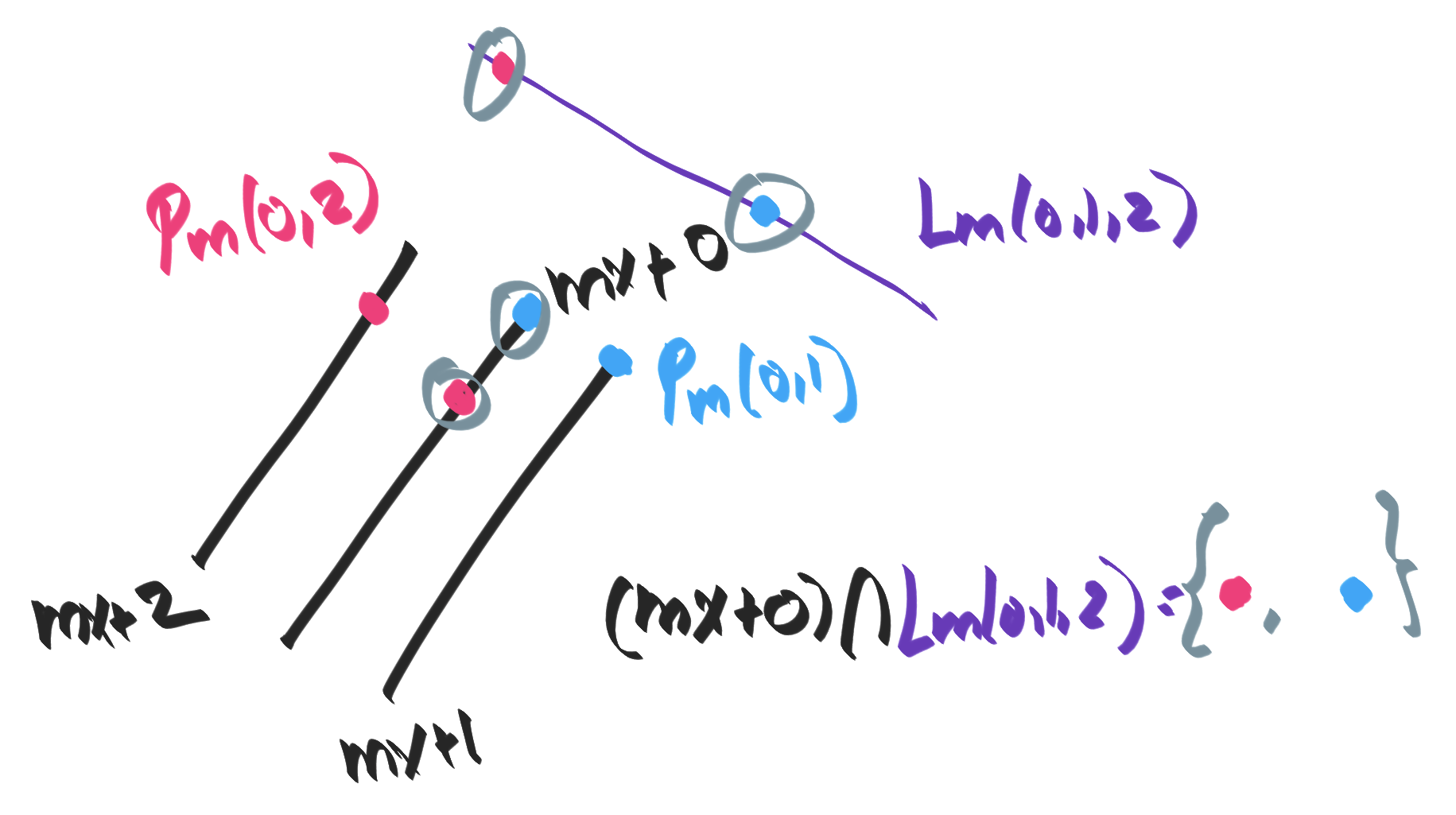## § Decomposition of projective space

Projective space $\mathbb P^{n+1} = \mathbb P^n \cup \mathbb R^n$. The current way I think about this is as follows (specialize to $n=3$)
• Consider a generic point $[x : y : z]$. Either $x = 0$ or $x \neq 0$.
• If $x = 0$, then we have $[0 : y : z]$ which can be rescaled freely: $[0: y: z] = (0, y, z, 1) = (0, \lambda y, \lambda z, \lambda) = [0: y: z]$. So, we get a component of $\mathbb P^2$ from the $[y: z]$.
• If $x \neq 1$, we have $[x : y : z]$. Spend the projectivity to get $(1:y:z) = (x, y, z, x)$. Now we have two free parameters, $(y, z) \in \mathbb{R^2}$. This gives us the $\mathbb R^2$.
There's something awkward about this whole thing, notationally speaking. Is there a more natural way to show that we have spent the projectivity to renormalize $[x: y: z]$ to $(1, y, z)$ ?

#### § Projective plane in terms of incidence

We can define $\mathbb P^2$ to be an object such that:
1. Any two lines are incident at a single point.
2. Two distinct points must be incident to a single line. (dual of (1))

#### § The points at infinity

This will give us a copy of $\mathbb R^2$, along with "extra points" for parallel lines.
• Consider two parallel lines $y = mx + 0$ and $y = mx + 1$. These don't traditionally meet, so let's create a point at infinty for them, called $P_m(0, 1)$.
• Now consider two more parallel lines, $y = mx + 0$ and $y = mx + 2$. These don't traditionally meet either, so let's create a point at infinite for them, called $P_m(0, 2)$.
• Finally, create another point $P_m(0, 3)$ as the point of intersection between $y = mx + 0$ and $y = mx + 3$.
• Now, consider $P_m(0, 1), P_m(0, 2), P_m(0, 3), dots$. We claim that they must all be equivalent. Assume not. Say that $P_m(0, 1) \neq P_m(0, 2)$.
• Then there must a line that joins $P_m(0, 1)$ an $P_m(0, 2)$. Call it $L_m(0, 1, 2)$. Now, what is the intersection between $L_m(0, 1, 2)$ and the line $y = mx + 0$? The points $P_m(0, 1)$ and $P_m(0, 2)$ both lie on the line $L_m(0, 1, 2)$. But this is a contradiction: two lines must be incident at a single unique point.
• So we must have $P_m(0, 1) = P_m(0, 2) = P_m$. So, for each direction, we must have a unique point where all lines in that direction meet.
We can make a definition: the point at infinity for a given direction is the equivalence class of all lines in that direction.#### § The line at infinity

This now begs the question: what lines to different points at infinity lie on? Let's consider $P_q, P_r, P_s, P_t$ as four points at infinity for four different slopes.
• Consider the lines $L(q, r)$ that is incident on $P_q$ and $P_r$, and then the line $L(s, t)$ that is incident on the lines $P_s$ and $P_t$.
• This begs the question: where do these lines meet? If we say that the meet at more new points of intersection, like $P(q, r, s, t)$ this process will never end.
• So we demand that all points at infinity lie on a unique line at infinity.